Next: The choice of Skyrme Up: The model Previous: The model

## Isospin- and angular-momentum-projected DFT approach

The building block of the isospin- and angular-momentum-projected DFT approach employed in this study is the self-consistent deformed MF state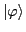that violates both the rotational and isospin symmetries. While the rotational invariance is of fundamental nature and is broken spontaneously, the isospin symmetry is violated both spontaneously and explicitly by the Coulomb interaction between protons. The strategy is to restore the rotational invariance, remove the spurious isospin mixing caused by the isospin SSB effect, and retain only the physical isospin mixing due to the electrostatic interaction [23,24]. This is achieved by a rediagonalization of the entire Hamiltonian, consisting the isospin-invariant kinetic energy and Skyrme force and the isospin-non-invariant Coulomb force, in a basis that conserves both angular momentum and isospin.

To this end, we first find the self-consistent MF stateand then build a normalized angular-momentum- and isospin-conserving basis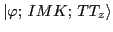by using the projection method: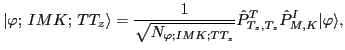(4)

where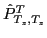and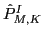stand for the standard isospin and angular-momentum projection operators: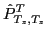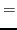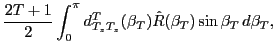(5)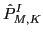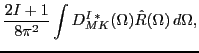(6)

where,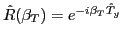is the rotation operator about the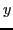-axis in the isospace,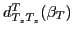is the Wigner function, and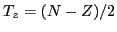is the third component of the total isospin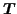. As usual,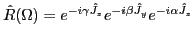is the three-dimensional rotation operator in space,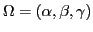are the Euler angles,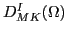is the Wigner function, and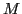and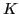denote the angular-momentum components along the laboratory and intrinsic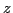-axis, respectively [22,25]. Note that unpaired MF statesconserve the third isospin component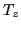; hence, the one-dimensional isospin projection suffices.

The set of states (4) is, in general, overcomplete because thequantum number is not conserved. This difficulty is overcome by selecting first the subset of linearly independent states known as collective space , which is spanned, for each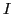and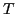, by the so-called natural states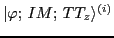[26,27]. The entire Hamiltonian - including the ISB terms - is rediagonalized in the collective space, and the resulting eigenfunctions are:(7)

where the index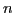labels the eigenstates in ascending order according to their energies. The amplitudes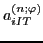define the degree of isospin mixing through the so-called isospin-mixing coefficients (or isospin impurities), determined for a giventh eigenstate as: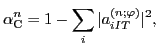(8)

where the sum of norms corresponds to the isospindominating in the wave function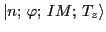.

One of the advantages of the projected DFT as compared to the shell-model-based approaches [28,3] is that it allows for a rigorous quantum-mechanical evaluation of the Fermi matrix element using the bare isospin operators: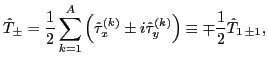(9)

where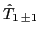denotes the rank-one covariant one-body spherical-tensor operators in the isospace, see the discussion in Ref. [29,30]. Indeed, noting that each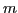th eigenstate (7) can be uniquely decomposed in terms of the original basis states (4),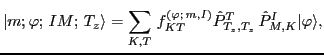(10)

with microscopically determined mixing coefficients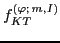, the expression for the Fermi matrix element between the parent state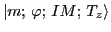and daughter state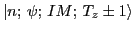can be written as: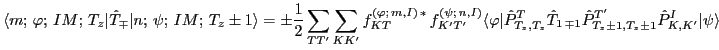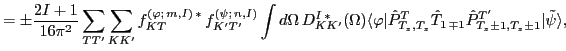(11)

where tilde indicates the Slater determinant rotated in space: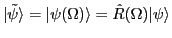. The matrix element appearing on the right-hand side of Eq. (11) can be expressed through the transition densities that are basic building blocks of the multi-reference DFT [31,32,33,24]. Indeed, with the aid of the identity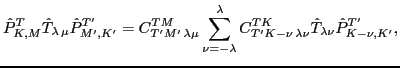(12)

which results from the general transformation rule for spherical tensors under rotations or isorotations,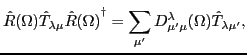(13)

the matrix element entering Eq. (11) can be expressed as: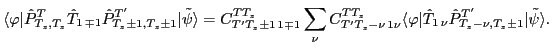(14)

For unpaired Slater determinants considered here, the double integral over the isospace Euler angles in Eq. (11) can be further reduced to a one-dimensional integral over the angle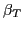using the identity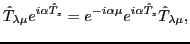(15)

which is the one-dimensional version of the transformation rule (13) valid for rotations around the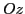axis in the isospace. The final expression for the matrix element in Eq. (14) reads: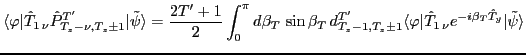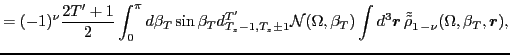(16)

where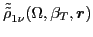is the isovector transition density, and the double-tilde sign indicates that the right Slater determinant used to calculate this density is rotated both in space as well as in isospace: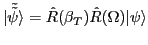. The symbol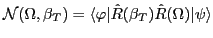denotes the overlap kernel.

Since the natural states have good isospin, the states (7) are free from spurious isospin mixing. Moreover, since the isospin projection is applied to self-consistent MF solutions, our model accounts for a subtle balance between the long-range Coulomb polarization, which tends to make proton and neutron wave functions different, and the short-range nuclear attraction, which acts in an opposite way. The long-range polarization affects globally all s.p. wave functions. Direct inclusion of this effect in open-shell heavy nuclei is possible essentially only within the DFT, which is the only no-core microscopic framework that can be used there.

Recent experimental data on the isospin impurity deduced in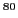Zr from the giant dipole resonance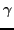-decay studies  agree well with the impurities calculated using isospin-projected DFT based on modern Skyrme-force parametrizations [23,17]. This further demonstrates that the isospin-projected DFT is capable of capturing the essential piece of physics associated with the isospin mixing.Next: The choice of Skyrme Up: The model Previous: The model
Jacek Dobaczewski 2012-10-19